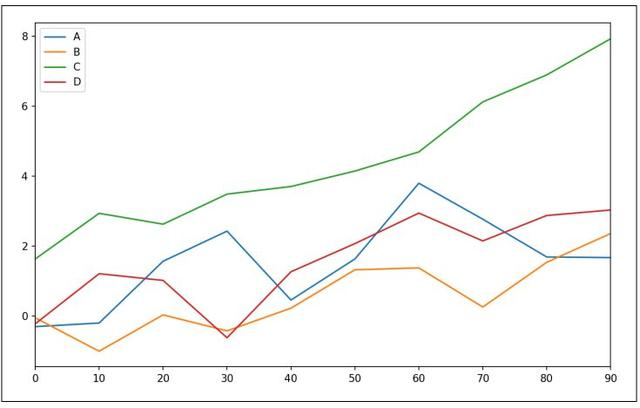# 使用pandas和seaborn制作炫酷图表：数据可视化干货

01 折线图

Series和DataFrame都有一个plot属性，用于绘制基本的图型。默认情况下，plot()绘制的是折线图(见图9-13)：

In : s = pd.Series(np.random.randn(10).cumsum(), index=np.arange(0, 100, 10))

In : s.plot()▲图9-13 简单序列图形

Series对象的索引传入matplotlib作为绘图的x轴，你可以通过传入use_index=False来禁用这个功能。x轴的刻度和范围可以通过xticks和xlim选项进行调整，相应地y轴使用yticks和ylim进行调整。表9-3是plot的全部选项列表。本节我会介绍这些选项中的一些，其余你可以自行探索。

DataFrame的plot方法在同一个子图中将每一列绘制为不同的折线，并自动生成图例(见图9-14)：

In : df = pd.DataFrame(np.random.randn(10, 4).cumsum(0),

....: columns=['A', 'B', 'C', 'D'],

....: index=np.arange(0, 100, 10))

In : df.plot()▲图9-14 简单DataFrame绘图

plot属性包含了不同绘图类型的方法族。例如，df.plot( )等价于df.plot.line( )。我们之后将会探索这些方法中的一部分。▲表9-3 Series.plot方法参数

DataFrame拥有多个选项，允许灵活地处理列；例如，是否将各列绘制到同一个子图中，或为各列生成独立的子图。参考表9-4了解更多选项。▲表9-4

02 柱状图

plot.bar()和plot.barh()可以分别绘制垂直和水平的柱状图。在绘制柱状图时，Series或DataFrame的索引将会被用作x轴刻度(bar)或y轴刻度(barh)(参考图9-15)：

In : fig, axes = plt.subplots(2, 1)

In : data = pd.Series(np.random.rand(16), index=list('abcdefghijklmnop'))

In : data.plot.bar(ax=axes, color='k', alpha=0.7)

Out: <matplotlib.axes._subplots.AxesSubplot at 0x7fb62493d470>

In : data.plot.barh(ax=axes, color='k', alpha=0.7)▲图9-15 水平柱状图和垂直柱状图

In : df = pd.DataFrame(np.random.rand(6, 4),

....: index=['one', 'two', 'three', 'four', 'five', 'six'],

....: columns=pd.Index(['A', 'B', 'C', 'D'], name='Genus'))

In : df

Out:

Genus A B C D

one 0.370670 0.602792 0.229159 0.486744

two 0.420082 0.571653 0.049024 0.880592

three 0.814568 0.277160 0.880316 0.431326

four 0.374020 0.899420 0.460304 0.100843

five 0.433270 0.125107 0.494675 0.961825

six 0.601648 0.478576 0.205690 0.560547

In : df.plot.bar()▲图9-16 DataFrame柱状图

In : df.plot.barh(stacked=True, alpha=0.5)▲图9-17 DataFrame堆积柱状图

In : tips = pd.read_csv('examples/tips.csv')

In : party_counts = pd.crosstab(tips['day'], tips['size'])

In : party_counts

Out:

size 1 2 3 4 5 6

day

Fri 1 16 1 1 0 0

Sat 2 53 18 13 1 0

Sun 0 39 15 18 3 1

Thur 1 48 4 5 1 3

# 没有太多的1人和6人派对

In : party_counts = party_counts.loc[:, 2:5]

# 标准化至和为1

In : party_pcts = party_counts.div(party_counts.sum(1), axis=0)

In : party_pcts

Out:

size 2 3 4 5

day

Fri 0.888889 0.055556 0.055556 0.000000

Sat 0.623529 0.211765 0.152941 0.011765

Sun 0.520000 0.200000 0.240000 0.040000

Thur 0.827586 0.068966 0.086207 0.017241

In : party_pcts.plot.bar()▲图9-18 每天派对数量的百分比

In : import seaborn as sns

In : tips['tip_pct'] = tips['tip'] / (tips['total_bill'] - tips['tip'])

In : tips.head()

Out:

total_bill tip smoker day time size tip_pct

0 16.99 1.01 No Sun Dinner 2 0.063204

1 10.34 1.66 No Sun Dinner 3 0.191244

2 21.01 3.50 No Sun Dinner 3 0.199886

3 23.68 3.31 No Sun Dinner 2 0.162494

4 24.59 3.61 No Sun Dinner 4 0.172069

In : sns.barplot(x='tip_pct', y='day', data=tips, orient='h')▲图9-19 用错误栏按天显示小费百分比

seaborn中的绘图函数使用一个data参数，这个参数可以是pandas的DataFrame。其他的参数则与列名有关。因为day列中有多个观测值，柱子的值是tip_pct的平均值。柱子上画出的黑线代表的是95%的置信区间(置信区间可以通过可选参数进行设置)。

seaborn.barplot拥有一个hue选项，允许我们通过一个额外的分类值将数据分离：

In : sns.barplot(x='tip_pct', y='day', hue='time', data=tips, orient='h')▲图9-20 根据星期几数值和时间计算的小费百分比

In : sns.set(style="whitegrid")

03 直方图和密度图

In : tips['tip_pct'].plot.hist(bins=50)▲图9-21 小费百分比的直方图

In : tips['tip_pct'].plot.density()▲图9-22 小费百分比密度图

distplot方法可以绘制直方图和连续密度估计，通过distplot方法seaborn使直方图和密度图的绘制更为简单。作为例子，考虑由两个不同的标准正态分布组成的双峰分布(见图9-23)：

In : comp1 = np.random.normal(0, 1, size=200)

In : comp2 = np.random.normal(10, 2, size=200)

In : values = pd.Series(np.concatenate([comp1, comp2]))

In : sns.distplot(values, bins=100, color='k')▲图9-23 正态混合的标准化直方图与密度估计

04 散点图或点图

In : macro = pd.read_csv('examples/macrodata.csv')

In : data = macro[['cpi', 'm1', 'tbilrate', 'unemp']]

In : trans_data = np.log(data).diff().dropna()

In : trans_data[-5:]

Out:

cpi m1 tbilrate unemp

198 -0.007904 0.045361 -0.396881 0.105361

199 -0.021979 0.066753 -2.277267 0.139762

200 0.002340 0.010286 0.606136 0.160343

###### 动态交互

© 2019 ESENSOFT 北京亿信华辰软件有限责任公司| 版权所有：京ICP备07017321号 京公网安备11010802016281号|免责声明

QQ：400-0011-866

(工作日9:00-18:00)